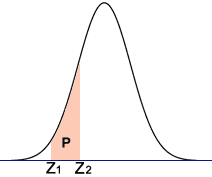# Calculating Defect Rate

• B
• rede96

#### rede96

TL;DR Summary
Need help to calculate the predicted defects per million for a given mean, standard deviation and specification limits.
Hi All

Assuming normal distribution I am trying to understand how to calculate the expected number of defects I would get from given specification limits.

For example assume a process mean of 51 and a standard deviation of 0.75 with a lower specification limit of 48.5 and a higher specification limit of 51.5

How do I calculate the lower and upper defects that would fall outside of the specification limits?

Would appreciate any help. Thanks!

Convert all numbers to the scale of a standard normal by subtracting the mean and dividing by the standard deviation. (e.g. 48.5 -> (48.5-50)/0.75 = -2.0) Then look up the cumulative probabilities for the lower and upper limits. That will allow you to determine the probability of a defect lying outside the limits.

CORRECTION: I used the wrong value for the mean. It should be 48.5 -> (48.5-51)/0.75 = -3.33333

Last edited:
Convert all numbers to the scale of a standard normal by subtracting the mean and dividing by the standard deviation. (e.g. 48.5 -> (48.5-50)/0.75 = -2.0) Then look up the cumulative probabilities for the lower and upper limits. That will allow you to determine the probability of a defect lying outside the limits.

Is there a way to do this just with summarised data? That is just with knowing the mean, standard deviation and specification limits?

Also could you explain what you mean by look up the cumulative probabilities for the lower and upper limits please?

Sorry haven’t done this for years.

Yes, if you use something like this

https://measuringu.com/calculators/pcalcz/

The -2 @FactChecker computed is called a z score. It's how many standard deviations above or below the mean your threshold is. The website helpfully shades the area under the graph it's computing to help you visualize what it's doing.

•FactChecker
Yes, if you use something like this

https://measuringu.com/calculators/pcalcz/

The -2 @FactChecker computed is called a z score. It's how many standard deviations above or below the mean your threshold is. The website helpfully shades the area under the graph it's computing to help you visualize what it's doing.
Thanks for the reply. I am still a little confused as I want to be able to work all this out myself.

To calculate the Z score I believe the formula is:

Zupper = (USL - Mean) / std deviation
Zlower = (Mean - LSL) / std deviation

For the data in my OP (mean 51, std deviation of 0.75, USL 51.5, LSL 48.5) that equates to:

Zupper = 0.667
Zlower = 3.333

So I now want to look up the Z scores to find the probability of defects outside of the lower and upper spec limits. And this is where I get confused.

I am using the NORMSDIST formula in Excel. Everything I read on the net says it should be just NORMSDIST(z) for the lower and 1-NORMSDIST for the upper.

But to get it to work correctly I have to do 1-NORMSDIST(z) for both the lower and the upper Z scores.

I would have thought the Zlower would have been negative, then I wouldn't need to do 1-NORMSDIST(z). I get why I have to do it for the Upper as I am looking to the right and the NORMSDIST works out the probability to the left.

Can anyone see where I am going wrong?

Is there a way to do this just with summarised data? That is just with knowing the mean, standard deviation and specification limits?
There are online calculators and tables to get the probabilities. (The probabilities are the areas under the normal bell-shaped curve and are not very simple.)
You can use this web page.
1) First, calculate your two z-scores: 48.5 -> -3.33333 and 51.5 -> 0.66666
2) Then, lower on the same web page, use those two z-scores to get that P( -3.3333<x<0.6666)=0.74708
3) Finally, since you are interested in the opposite probability, where the value is not between those z-scores, subtract from 1 to get 1-0.74708 = 0.25292
Also could you explain what you mean by look up the cumulative probabilities for the lower and upper limits please?
The web page has diagrams that illustrate the situation (but for your question, you want the part under the curve that is NOT shaded)Last edited:
Thanks for the reply. I am still a little confused as I want to be able to work all this out myself.

To calculate the Z score I believe the formula is:

Zupper = (USL - Mean) / std deviation
Zlower = (Mean - LSL) / std deviation
No. Zlower = (LSL - Mean) / std deviation
For the data in my OP (mean 51, std deviation of 0.75, USL 51.5, LSL 48.5) that equates to:

Zupper = 0.667
Zlower = 3.333
This is wrong. Zlower = -3.333

CORRECTION: I used the wrong value for the mean. It should be 48.5 -> (48.5-51)/0.75 = -3.33333

Ah ok, I didn't spot that either.

So is Zlower always going to be:
(LSL - mean) / std deviation
and Zupper always going to be:
(USL - mean)/std deviation

•FactChecker